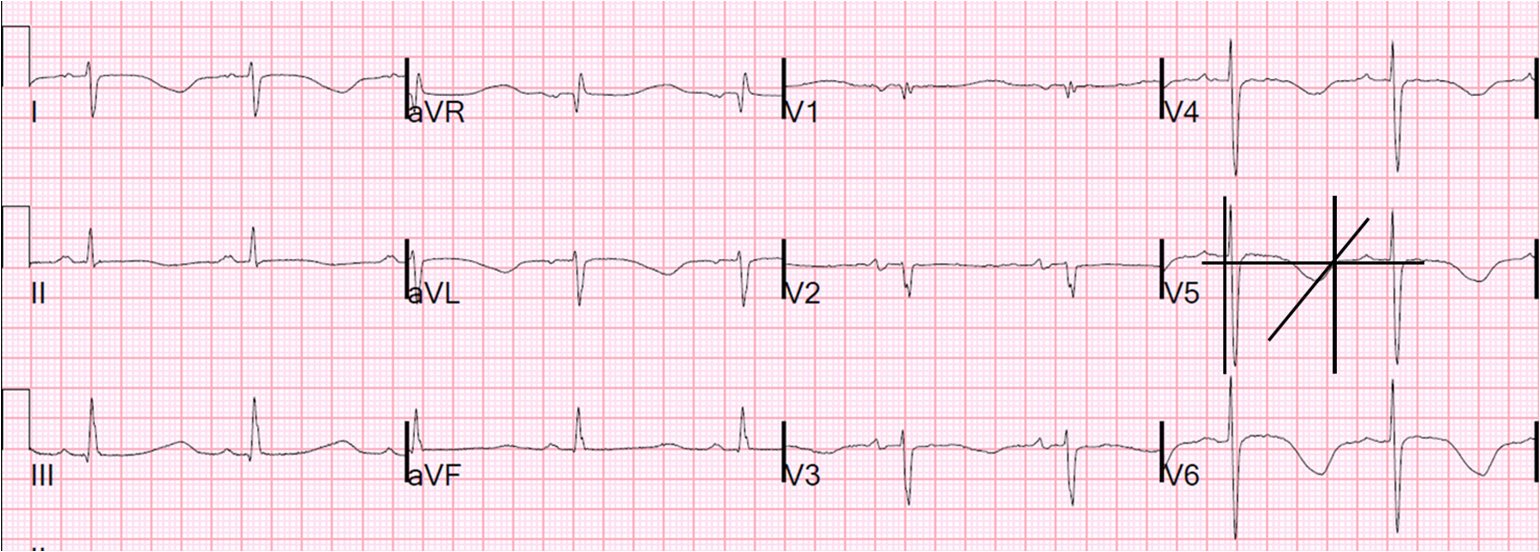## Tuesday, June 3, 2014

### Long QT: Do not trust the computerized QT interval when the QT is long

A middle-aged male with h/o DM was found down.  He was hypoglycemic (mild, 45 mg/dl) and had pneumonia with hypoxia.  He had this ECG recorded:Sinus rhythm with slight right axis deviation and non-diagnostic T-wave inversions

He received azithromycin and ceftriaxone for community acquired pneumonia.  Then he became very agitated in spite of correction of hypoglycemia, and was given a total of 15 mg of haloperidol.  His K returned at 2.8 mEq/L and ionized Calcium at 3.82 mEq/L (normal, 4.4 -5.2).  Magnesium was 1.5 mEq/L (normal, 1.3 - 2.0)

A troponin returned slightly elevated, so another ECG was recorded:There is now sinus bradycardia with a bizarrely long QT interval.  The computer read the QT as 450 ms when it is really around 700 ms.

This is a very dangerously long acquired long QT, and is due to a combination of azithromycin, haloperidol, hypokalemia, and hypocalcemia (note the long ST segments which are typical of hypocalcemia).

He was given Magnesium, Calcium, and Potassium, monitored in the ICU, and the eventually the ECG corrected as the drugs metabolized.

An echocardiogram showed no wall motion abnormality and the elevated troponin was not thought to be due to a Type 1 MI in this critically ill patient.

Comment: computer and manual QT measurement

Computer algorithms for measure the QT interval are good for normal QT intervals but not for long QT intervals, and are particularly inaccurate for very long QT intervals.

The QT is the interval from the beginning of the QRS to the end of the T-wave.  It should be the longest QT interval of all 12 leads, and this is the way most computer algorithms measure it.  However, taking the longest of leads II, V2, V5, and V6 will usually do.

One does not actually measure the end of the T-wave: instead, the technique involves drawing a line along the maximum downslope of the T-wave and measuring from where it intersects with the isoelectric line.  Here is a graphic of this from Life in the Fast Lane (same as the graphic in this link).

Once you measure the QT, then the most commonly used heart rate correction is the Bazett formula, which is the QT divided by the square root of the preceding R-R interval (example: if the preceding R-R interval is 810 ms = 0.81 sec, then the square root of 0.81 is 0.9, so a QT interval of 450 ms would result in a QTc of 500 ms (450 divided by 0.9 = 500).

Let's measure the QT on the above tracing:Lead V5 is the most convenient and appears to be as long as any other lead.  The first vertical line is the start of the QRS.  The angled line is on the steepest part of the T-wave slope.  The horizontal line is the isoelectric line.  The intersection of the angled line and the isoelectric is where the second vertical line is.  Then the measurement is between the two vertical lines.  There are 3 large 5 mm boxes (each 200 ms) + 2.25 mm to the left and 1.25 mm to the right.  That is 18.5 mm x 40 ms per mm = 740 ms.  QTc = 740 divided by the square root of the R-R interval of 1.08 seconds = 740 divided by 1.04 = 711 ms.QTc = 711 ms

Life in the Fast Lane has an excellent overview of QT prolongation.

Acquired long QT, and how it causes torsade: It is usually due to drugs.  The list is long.  And also due to electrolyte abnormalities, especially hypoK and hypoMg.   Corrected QT interval (Bazett correction = QT divided by the square root of the preceding R-R interval in milliseconds) is usually great than 600 ms.  Torsades in acquired long QT is much more likely in bradycardia because the QT interval following a long pause is longer still.  Thus, torsades in acquired long QT is called "pause dependent": if there is a sinus beat after a long pause (which creates a longer QT interval), then an early PVC ("early afterdepolarization," EAD) is much more likely to occur during repolarization and to initiate torsades.  The usual sequence is: sinus beat, then early PVC, then a long pause because the PVC was early, which then results in a particularly long QT, then another PVC with "R on T" that initiates torsades.

See this case of Polymorphic Ventricular Tachycardia.

#### 1 comment:

1.